•matlab
• [数值积分]龙贝格公式求数值积分 梯形递推的变步长积公式虽然算法简单，但精度低，收敛的速度慢，因此，研究收敛速度快、精度高的龙贝格算法显得尤为重要。 例：用龙贝格算法f(x) = sin(x) / x 在区间[0,1]的...
梯形递推的变步长求积公式虽然算法简单，但精度低，收敛的速度慢，因此，研究收敛速度快、精度高的龙贝格算法显得尤为重要。
例：用龙贝格算法求f(x) = sin(x) / x 在区间[0,1]的数值积分，精度要求为1e-6.

加工流程：程序框图设计：运行示例：源码：

#include<iostream>
#include<cmath>
double f(double x);   //自定义函数

using namespace std;

int main(void)
{
double a, b, accuracy;   //a为区间下限，b为区间上限，accuracy为精度

cout << "请输入积分区间:";   //输入提示
cin >> a >> b;

cout << "请输入精度:";   //输入提示
cin >> accuracy;

double h;   //步长

h = b - a;

double T1, T2;   //T1：二分前的梯形法积分值；T2：二分后的梯形法积分值；

T1 = h / 2 * (1 + f(b));
T2 = 0;

int k = 1;  //记录二分次数

cout << "T" << pow(2, 0) << " = " << T1 << endl;

double S1, S2;   //对T1与T2加权平均，得辛普森积分值
double C1, C2;   //对S1与S2加权平均，得柯特斯积分值
double R1, R2;   //对C1，C2加权平均，得龙贝格积分值
int flag = 1;   //flag作为循环控制标志性变量

while (flag == 1)
{
double sum = 0;  //各分点的函数值和
double x = a + h / 2;   //分点值

while (x < b)   //在区间上限范围内求各分点的函数值和
{
sum += f(x);
x += h;
}

T2 = T1 / 2 + h / 2 * sum;    //计算梯形序列得下一个二分结果
cout << "T" << pow(2, k) << " = " << T2 << endl;

S2 = T2 + 1.0 / 3 * (T2 - T1);   //线性组合外推值simpson
cout << "S" << pow(2, k - 1) << " = " << S2 << endl;

if (k == 1)   //至少外推2次得出S1，S2
{
k++;
h /= 2;
T1 = T2;
S1 = S2;
continue;
}
else
{
C2 = S2 + 1.0 / 15 * (S2 - S1);  //线性组合外推值Cotes
cout << "C" << pow(2, k - 2) << " = " << C2 << endl;

if (k == 2)   //至少外推3次得出C1，C2
{
C1 = C2;
k++;
h /= 2;
T1 = T2;
S1 = S2;
continue;
}
else
{
R2 = C2 + 1.0 / 63 * (C2 - C1);   //线性组合外推至Romberg
cout << "R" << pow(2, k - 3) << " = " << R2 << endl;

if (k == 3)   //至少外推4次得出R1,R2
{
R1 = R2;
C1 = C2;
k++;
h /= 2;
T1 = T2;
S1 = S2;
continue;
}
else if (abs(R2 - R1) >= accuracy)   //精度仍然不符合要求，继续二分步长、继续外推
{
R1 = R2;
C1 = C2;
k = k + 1;
h = h / 2;
T1 = T2;
S1 = S2;
}
else   //精度符合要求，修改flag为0，跳出while循环
{
flag = 0;
cout << "Romber算法求得数值积分结果为:" << R2 << endl;
}
}
}
}

return 0;
}

double f(double x)   //自定义被积函数
{
double result;

result = sin(x) / x;

return result;
}



展开全文c++
• 查找根法 给定一个闭区间，我们假设函数f(x)在该区间内有且仅有一个零点，则令f(x) = 0，在该区间内不断折半区间，根据有根的判别条件f(x1) x f(x2) < 0,则经过不断地折半，我们总能找到一个给定了精度的根...
给定一个闭区间，我们假设函数f(x)在该区间内有且仅有一个零点，则令f(x) = 0，在该区间内不断折半区间，根据有根的判别条件f(x₁) × f(x₂) < 0,则经过不断地折半，我们总能找到一个给定了精度的根，并且有理由相信，该根x关于精度是“准确”的。在实际操作过程中，我们用在精度控制下找到有根区间的中点的值作为方程的近似解。
示例：用二分法求方程x³ - x - 1 = 0 在区间[1,1.5]内的一个实根，要求误差不超过0.005。

运行示例：源码：

//实现用二分查找求根法：求出给定精度给定方程的根
#include<iostream>
#include<cmath>
using namespace std;

//函数名：function
//参数：用户输入的值x
//返回值：输入参数值的函数值
//功能：求解给定值的函数值
double function(double x)   //用户自定义的函数，可修改
{
double result;
//求解自定义函数值的函数
result = pow(x, 3) - x - 1;
return result;
}

int main(void)
{
double low, up, mid=0, accuracy;    //区间上下限、中点、精度
int i=0;

cout << "请输入区间上、下限：";
cin >> low;
cin >> up;
cout << "请输入精度：";
cin >> accuracy;

cout << endl;

do
{

//区间取半
mid = (low + up) / 2;

//判断根的位置
if ((function(low) * function(mid)) < 0)    //根在前半区间
{
up = mid;   //将区间中点值赋值给区间上限
i++;    //折半查找次数自增1
cout << "第" << i << "次折半！" << endl ;
cout << "根位于前半区间！" << endl<<endl;
}
else   //根在后半区间
{
low = mid;   //将区间中点的值赋值给区间下限
i++;   //折半查找次数自增1
cout << "第" << i << "次折半！" << endl ;
cout << "根位于后半区间！" << endl << endl;
}
} while ((up - low) > accuracy);   //若i次二分区间后，区间长度小于精确度，则二分结束，找到近似根

cout << "解为：";  //用区间中点的值作为根的近似解
cout << mid << endl;

return 0;
}



展开全文c++
• 然而，微分方程大多数并不能直接出解析式，因此在求解微分方程时更多采用数值计算。 1.2 数值求解微分方程的基本思路是将时间和空间离散化，然后将微分转化为差，推导出差递推式，从而利用边界条件和初始条件...
1. 引言
1.1微分方程是描述一个系统的状态随时间和空间变化的最基本的数学表达式，在物理，经济，工程等方面都有及其重要的作用。然而，微分方程大多数并不能直接求出解析式，因此在求解微分方程时更多采用数值计算。
1.2 数值求解微分方程的基本思路是将时间和空间离散化，然后将微分转化为差分，推导出差分递推式，从而利用边界条件和初始条件进行求解。
2 常微分方程的差分求解
一般的，一阶微分方程为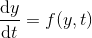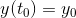首先将连续变量y和t离散化，连续的函数y(t)和f(y,t)化为离散的
$y_{_{n}}$和$f(y_{n},t_{n})$,上述微分方程可以化为差分方程
$\frac{y_{n+1}-y_{n}}{\Delta t}=\frac{f(y_{n},t_{n})+f(y_{n+1},t_{n+1})}{2}$
$y(0)=y_{0}$
从而我们得到递推关系
$y_{n+1}=y_{n}+\frac{\Delta t}{2}(f(y_{n},t_{n})+f(y_{n+1},t_{n+1}))$
$y(0)=y_{0}$
有了递推关系和初始条件就可以利用python 强大的计算能力，得到任意的$y_{n}$
3 RC回路的放电问题
对于一个$RC$回路，我们有
$IR+\frac{Q}{C}=0$
其中，$I,R,Q,C$分别为电流，电阻，电量和电容，利用$I=\frac{dQ}{dt}$,并定义$\tau \equiv RC$，我们得到一个含初始条件的一阶常微分方程
$\frac{dQ}{dt}=-\frac{Q}{\tau}$
$Q(t_{0})=Q_{0}$
这个方程当然可以解析求解，得到$Q=Q_{0}e\tfrac{-t}{\tau }$。另一方面按照差分法，可以得到递推关系式
$Q_{n+1}=Q_{n}-\frac{1}{2}(\frac{Q_{n}}{\tau}+\frac{Q_{n+1}}{\tau})\Delta t$
$Q(0)=Q_{0}$
下面我们用python进行数值求解，并和解析解进行比较
import numpy as
import matplotlib.pyplot  as plt
rc=2.0 #设置常熟=数
dt=0.5#设置步长
n=1000#设置分割段数
t=0.0#初始时间
q=1.0#设置初始电量

#先定义三个空列表
qt=[]#用来盛放差分得到的q值
qt0=[]#用来盛放解析解得到的q值
time=[]#用来放时间
for i in range(n):
t=t+dt
q1=q-q*dt/rc  #qn+1的近似值
q=q-0.5*(q1*dt/rc+q*dt/rc)
q0=np.exp(-t/rc)#解析关系
qt.append(q)
qt0.append(q0)
time.append(t)o
plt.plot(time,qt,'o',label='Euler_Modify')
plt.plot(time,qt0,'r-',label='Analytcal')
plt.xlabel('time')
plt.ylabel('charge')
plt.legend()
plt.show()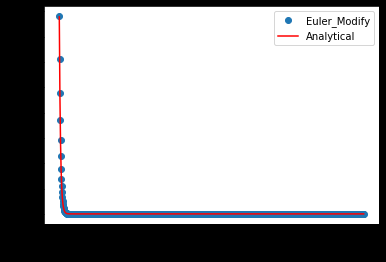下一篇将介绍一维偏微分方程求解。


展开全文python 算法
• 文章目录实验目标编程语言与扩展库二分法根牛顿法根弦截法根写在最后 实验目标 用编程语言编程实现以下...# 二分求根法 求解f(x)=0 def Dicphoni(a,b,tol): # 初始值 y0 = f(a) # 迭代直到区间小于tol while
• matlab求解偏微分方程，相比较把偏微分转成长分为方程再调用ode函数，利用离散差法，使用迎风格式迭代求解数值解。matlab pde
• 数值积分之Newton-Cotes积公式1. Newton-Cotes积公式1.1 N-C积公式的推导1.2 N-C积公式的余项1.3 N-C积公式的数值稳定性2. 复合积公式2.1 复合梯形积公式2.2 复合Simpson积公式 1. Newton-Cotes积...
数值积分之Newton-Cotes求积公式和复合求积公式1. Newton-Cotes求积公式1.1 N-C求积公式的推导1.2 N-C求积公式的余项1.3 N-C求积公式的数值稳定性2. 复合求积公式2.1 复合梯形求积公式2.2 复合Simpson求积公式
1. Newton-Cotes求积公式
1.1 N-C求积公式的推导
在《数值分析(8)：数值积分之Lagrange法》中已经介绍了插值型求积公式，它是用n次lagrange插值多项式来近似被积函数，从而对原积分进行近似（因为原积分被积函数的原函数不一定能写出来，而多项式函数的原函数一定能写出来）
被积函数用n次lagrange插值多项式替代就涉及到如何选取n+1个插值节点的问题，如果将被积区间等间隔地分为n份，从而得到n+1个插值节点，那么这样得到的求积公式称为Newton-Cotes求积公式。
N-C求积公式的推导如下：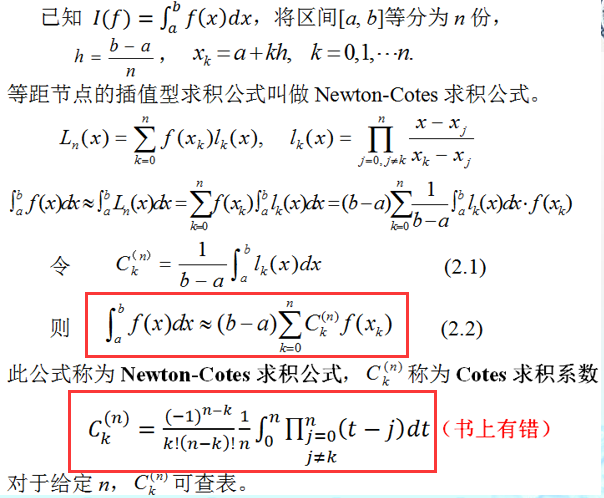可以看到使用N-C求积公式时，最难求的是$C^{(n)}_k$，而$f(x_k)$是很容易求出来的。注意到$C^{(n)}_k$是与被积函数$f(x)$和求积区间 无关的，它只与 节点个数$n$与求和项$k$有关，因此可以列出如下的$C^{(n)}_k$的表：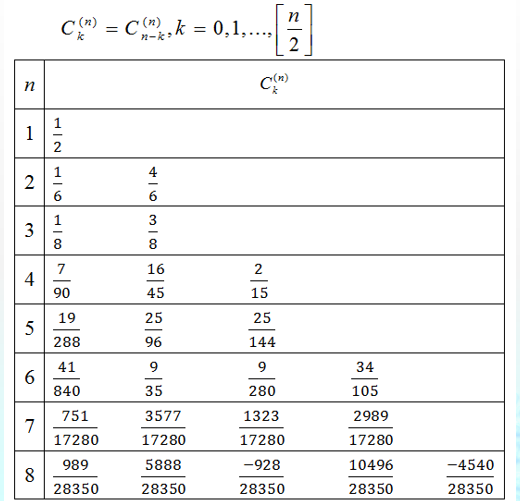注意$C^{(n)}_k$的值具有与组合数类似的对称性
1.2 N-C求积公式的余项
很自然我们想要知道N-C求积公式的近似误差是多少？也就是求积余项，或者说代数精度。对此，有如下定理：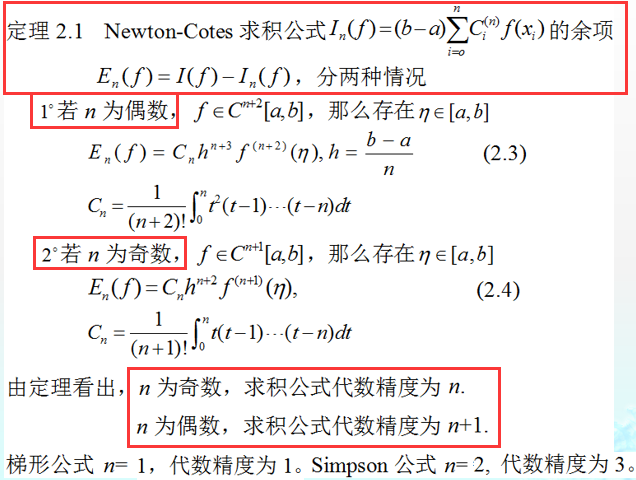1.3 N-C求积公式的数值稳定性
关于数值稳定性的定义可以见《数值分析(1)：数学模型和数值方法引论》
简单来说，如果初始数据的微小改变会引起最后结果的巨大改变，那么就称作是数值不稳定的，反之则称为数值稳定的。
对于求积公式的数值稳定性有如下定义：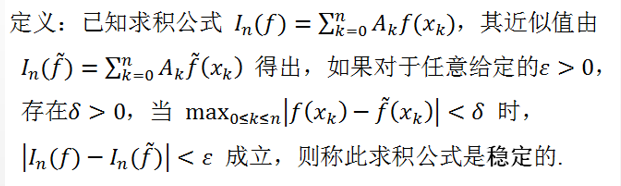因为在计算$f(x_k)$时可能存在误差，所以有必要分析N-C求积公式的数值稳定性。对于N-C求积公式的数值稳定性有如下推导和定理：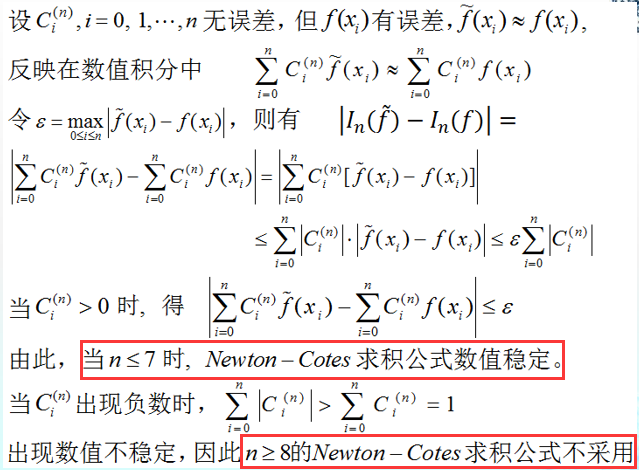2. 复合求积公式
在前面的N-C求积公式分析中可以知道，采用等分区间选节点的方式来计算lagrange多项式，从而近似积分有缺陷，如果节点选少了，势必会导致近似误差大，如果节点选多了，一旦超过7个节点，那么是数值不稳定的。
如何解决这一困境？
解决方式就是：把整个积分区间分成若干个子区间（通常是等分)，再在每个子区间上采用低阶求积公式，这种方法称为复合求积方法。
复合求积方法是每个区间就有一个lagrange插值多项式函数。根据插值多项式的次数不同，可以分为：复合梯形求积公式、复合Simpson求积公式
2.1 复合梯形求积公式
复合梯形求积公式的推导如下：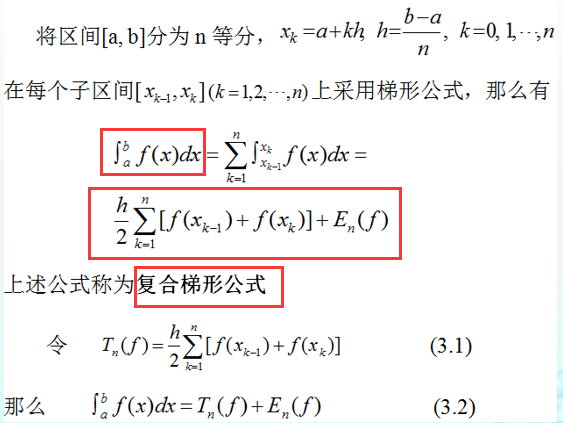当然，因为区间是等分的，所以$T_n(f)$还可以用下式计算：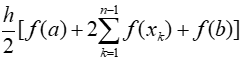再看复合梯形求积公式的余项：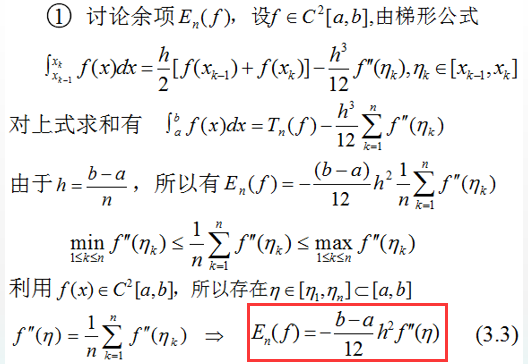最后看复合梯形求积公式的收敛性：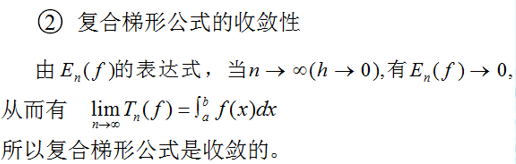2.2 复合Simpson求积公式
复合Simpson求积公式的推导如下：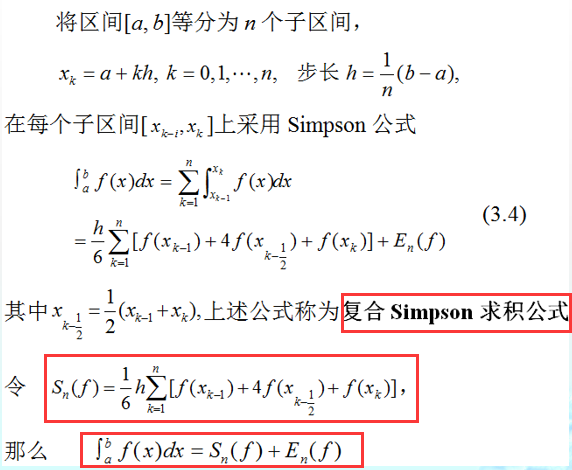同样因为区间是等分的，所以$S_n(f)$的表达式可以化简为：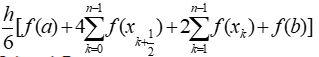再看复合Simpson求积公式的余项：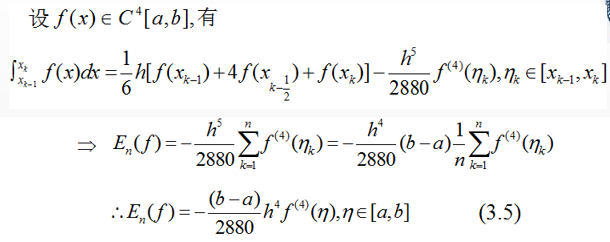最后看复合Simpson求积公式的收敛性：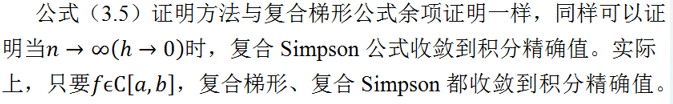复合梯形求积公式和复合Simpson求积公式必定收敛这个事情是显然的，因为定积分的切割法其实就是“复合矩形求积公式”，那么精确度更高的复合梯形求积公式和复合Simpson求积公式自然也会收敛。
参考文献：
关治，陆金甫《数值方法》


展开全文• 我们如何从有限的点集（波形或曲线）得到...一般我们有三种近似法，前向差、后向差和中心差，中心差误差最小。具体信息见参考资料。下面是三种方法定义的截图。 参考资料 Numerical Differentiation ...
• 主要是数值分析_二_牛顿_ 复合梯形公式 复合 辛普森积公式的算法步骤
• Gauss型积公式1. 引言2. Gauss型积公式2.1 ...在前一章《数值分析(9)：数值积分之Newton-Cotes积公式和复合积公式》中，提出使用等分区间的方式来给出插值节点，从而得到lagrange插值多项式，最后得到Newton
• 数值方法π和π的x次方πlnππ的x次方重中之重：误差分析 学习了数值分析这门课，老师留了一个作业是设计数值方法π的x次方（x范围为1到10） 具体步骤如下（老师要求的，就是想让你多用用几种数值方法...误差分析 辛普森公式
• 学号 班级 统计1001 姓名 指导教师 易昆南 实验题目 用多种方法计算数值积分 评 1设计实习目的 了解MATLAB在实际问题中的应用 通过实践加深对这门语言中M文件的了解 熟悉简单程序结构如循环结构for循环while循环...
• 高斯积代码matlab ...牛顿除数差插值多项式和带有Chebyshev节点的多项式插值 数值积分 梯形法，辛普森法则，高斯数值线性代数 高斯消除，LU分解，SVD，迭代方法（Gauss-Seidel，连续过松弛，Jacobi）
• 用于根据累积分布函数 (CDF) 确定位数和相关值的实用程序，该函数表示为数值向量和可选的数值权重向量。 可以将位数向量转换为关联 CDF 值的向量。 也可以将 CDF 值的向量转换为相关的位数。matlab
• 变步长辛普森积算法，是计算数值积分里面的一个常用算法。与变步长梯形公式一样，将积区间逐步二，在每个子区间上运用辛普森公式，最后累加起来。与变步长梯形公式相比，该算法就是运算次数较少，比复合梯形...算法
• 用牛顿(Newton)迭代法求分f(x)=0在x0附近的根 首先引自百度百科的牛顿迭代法的定义： 算法描述: (1)任取迭代初始值X0 (2)计算x1= x0-f’(x0)/f’(x0); (3)判断收敛性:如果|x1 -x0|<ε或者|f(x1)|<ε 则算法...经验分享 程序人生
• 利用Richardson外推算法，得到如下的积方法，其只产生四个序列：...有一定数值分析基础，不难写出如下程序 #include #include #include #include using namespace std; ///#define f(x) (sin(x)) double f(dou
• 数值微分与数值积分数值微分数值积分（3）...MATLAB提供了向前差的函数diff，其调用格式有三种：  dx=diff(x)：计算向量x的向前差，dx(i)=x(i+1)-x(i)，i=1，2，…，n-1。  dx=diff(x,n)：计算向量x的n阶向matlab
• 为了能适合更多的f(x)，我们一般使用牛顿-科特斯公式其中比较高次的公式来进行数值求积。但高次的缺陷是当次数大于8次，积公式就会不稳定。因此，我们用于数值积分的牛顿-科特斯公式通常是一次的梯形...python
• 方程根 二搜索 步骤： 从所给区间[a,b]着手二，令a1<=a,b1<=b 取有根区间[a1,b1]的中点x作为近似根 通过根的搜索确定二后新的有根区间[a1,b1] 检查近似根x是否满足精度要求：若不满足则转步2继续二...二分法 算法 机器学习
• 在0-一个数值之间的随机取值我们经常会碰见,但是如果说在俩个数值之间随机取值可能,炸一听到,就愣一下,其实吧 仔细思考下也不难哈 比如举个例子吧 :10 到 20至今随机去数字 Math.random()*10 [0,10) Math.random()*...
• 数值微分意义： 前向差公式 后向差公式 两点中心差公式（一阶导数近似、二阶导数近似） Richardson外推
• 高阶差格式的设计方法及数值计算，刘乐柱，，本文详细介绍了高阶差格式的构造方法，分别给出了用于一阶微商、二阶微商的高阶差格式及边界处理方法。应用传统差格式与
• 实现一个函数，返回二叉树bt中度为2的结点的数值之和(不是数量之和)！ 函数接口定义： <p>int sumDCNodes(struct BinTree *bt);</p>c# 有问必答
• 一． 实验目的 1. 熟悉使用MATLAB软件。 2. 了解数值计算微分的向前、中、后差公式。 3. 掌握数值计算中的各种积公式。...本实验主要是通过编程去实现数值计算中的一些积公式与差公式。
• 数值解，是指给出一系列对应的自变量，采用数值方法出的解。采用的方法有限元法、数值逼近、插值法。他人只能利用数值计算的结果，而不能随意给出自变量并出计算值。当无法由微积分技巧求得解析解时，便只能利用......